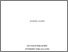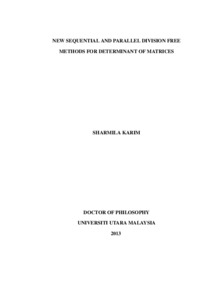UUM Electronic Theses and Dissertation
UUM ETD | Universiti Utara Malaysian Electronic Theses and Dissertation
FAQs | Feedback | Search Tips | Sitemap

# New Sequential and Parallel Division Free Methods for Determinant of Matrices

Sharmila, Karim (2013) New Sequential and Parallel Division Free Methods for Determinant of Matrices. PhD. thesis, Universiti Utara Malaysia.Preview
Text
s92168.pdf

## Abstract

A determinant plays an important role in many applications of linear algebra. Finding determinants using non division free methods will encounter problems if entries of matrices
are represented in rational or polynomial expressions, and also when floating point errors arise. To overcome this problem, division free methods are used instead. The
two commonly used division free methods for finding determinant are cross multiplication and cofactor expansion. However, cross multiplication which uses the Sarrus Rule only works for matrices of order less or equal to three, whereas cofactor expansion requires lengthy and tedious computation when dealing with large matrices. This research, therefore, attempts to develop new sequential and parallel methods for finding determinants of matrices. The research also aims to generalise the Sarrus Rule for any order of square matrices based on permutations which are derived using starter sets. Two strategies were introduced to generate distinct starter sets namely the circular and the exchanging of two elements operations. Some theoretical works and mathematical properties for generating permutation and determining determinants were also constructed to support the research. Numerical results indicated that the new proposed methods performed better
than the existing methods in term of computation times. The computation times in the newly developed sequential methods were dominated by generating starter sets. Therefore, two parallel strategies were developed to parallelise this algorithm so as to reduce the computation times. Numerical results showed that the parallel methods were able to
compute determinants faster than the sequential counterparts, particularly when the tasks were equally allocated. In conclusion, the newly developed methods can be used as viable alternatives for finding determinants of matrices.

Item Type: Thesis (PhD.) Ibrahim, Haslinda and Othman, Khairil Iskandar 3869 Determinant, Permutation, Starter Sets, Division Free Method, Sequential and Parallel Methods Q Science > QA Mathematics Awang Had Salleh Graduate School of Arts & Sciences 09 Mar 2014 02:44 25 Apr 2016 02:14 Awang Had Salleh Graduate School of Arts and Sciences Ibrahim, Haslinda and Othman, Khairil Iskandar https://etd.uum.edu.my/id/eprint/3869